## 配电网中性点接地补偿的单相逆变器接地系统原理与设计

Wang W, Yan L, Zeng X, et al. Principle and design of a single-phase inverter-based grounding system for neutral-to-ground voltage compensation in distribution networks[J]. IEEE Transactions on Industrial electronics, 2016, 64(2): 1204-1213.

### 2. 主动接地系统原理

#### A. 中性点接地电压补偿原理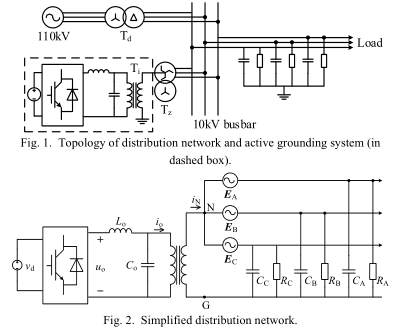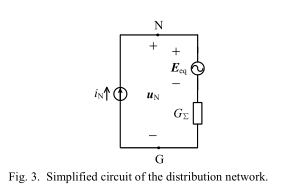$E _ { eq } = - G _ { \Sigma } ( E _ { A } Y _ { A } + E _ { B } Y _ { B } + E _ { C } Y _ { C } )\tag{1}$ $G _ { \Sigma } = \frac { 1 } { Y _ { \Sigma } } = \frac { 1 } { Y _ { A } + Y _ { B } + Y _ { C } }\tag{2}$

$u _ { N } = i _ { N } G _ { \Sigma } + E _ { eq } = G _ { \Sigma } ( i _ { N } - E _ { A } Y _ { A } - E _ { B } Y _ { B } - E _ { C } Y _ { C } )\tag{3}$

$i _ { 0 } = E _ { A } Y _ { A } + E _ { B } Y _ { B } + E _ { C } Y _ { C }\tag{4}$

$u _ { N } = G _ { \Sigma } ( i _ { N } e ^ { j \theta } - i _ { 0 } e ^ { j \theta _ { 0 } } )\tag{5}$

$u _ { N } ( i _ { N } , \theta ) = | G _ { \Sigma } | \sqrt { i _ { N } ^ { 2 } + i _ { 0 } ^ { 2 } - 2 i _ { N } i _ { 0 } \operatorname { cos } ( \theta - \theta _ { 0 } ) }\tag{6}$

$\frac { \partial u _ { N } ( i _ { N } , \theta ) } { \partial i _ { N } } = | G _ { \Sigma } | \frac { i _ { N } - i _ { 0 } \operatorname { cos } ( \theta - \theta _ { 0 } ) } { \sqrt { i _ { N } ^ { 2 } + i _ { 0 } ^ { 2 } - 2 i _ { N } i _ { 0 } \operatorname { cos } ( \theta - \theta _ { 0 } ) } }\tag{7}$ $\frac { \partial u _ { N } ( i _ { N } , \theta ) } { \partial \theta } = | G _ { L } | \frac { i _ { N } i _ { 0 } \operatorname { sin } ( \theta - \theta _ { 0 } ) } { \sqrt { i _ { N } ^ { 2 } + i _ { 0 } ^ { 2 } - 2 i _ { N } i _ { 0 } \operatorname { cos } ( \theta - \theta _ { 0 } ) } }\tag{8}$

$i _ { N } = i _ { 0 } \operatorname { cos } ( \theta ^ { * } - \theta _ { 0 } )\tag{9}$

$u_N$的变化趋势可以在几种不同的情况下进行讨论， 若$- \pi / 2 \leq \theta ^ { * } - \theta _ { 0 } < \pi / 2$，随着$i_N$从0增大到无穷大，$u_N$会先降后升。着意味着$u_N$由一个最小值。当$\pi / 2 \leq \theta ^ { * } - \theta _ { 0 } < 3 \pi / 2$，$u_N$会单调增加，所以，$u_N$也会由一个最小值。

$\theta = \theta_0\tag{10}$

#### B. 补偿电流检测方法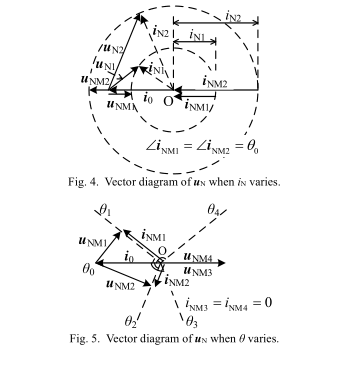### 3. 电流控制策略

#### A. 控制模型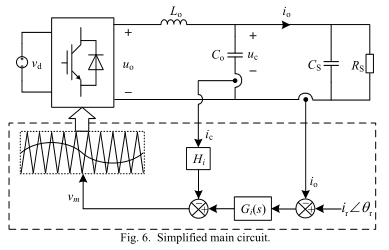$G _ { 1 } ( s ) = \frac { i _ { 0 } ( s ) } { v _ { m } ( s ) } = \frac { K _ { pwm } ( s R _ { s } C _ { s } + 1 ) } { s ^ { 2 } R _ { s } L _ { 0 } ( C _ { o } + C _ { s } ) + s L _ { o } + R _ { s } }\tag{11}$

$\omega _ { r } = \sqrt { \frac { 1 } { L _ { 0 } ( C _ { 0 } + C _ { s } ) } }\tag{12}$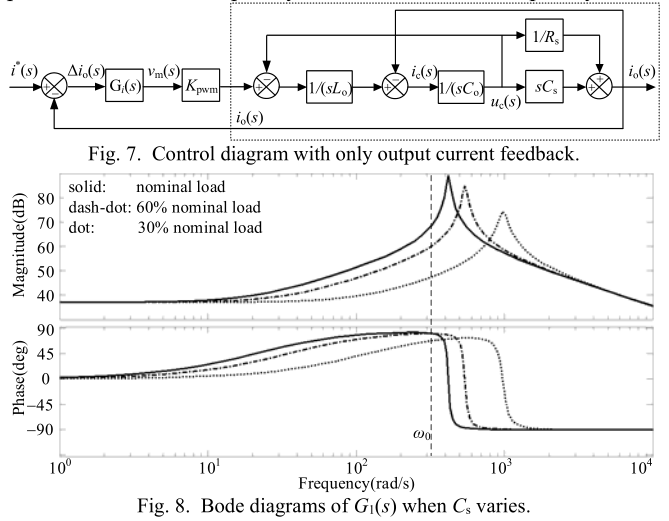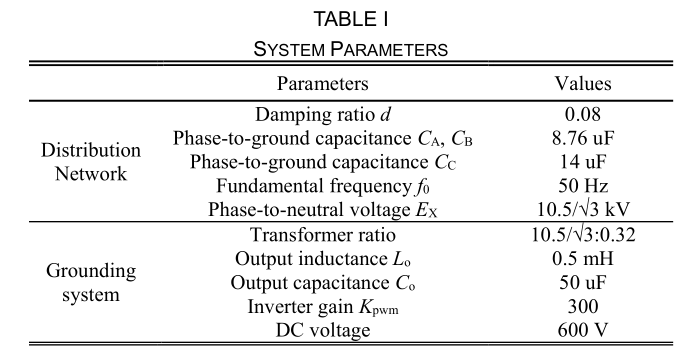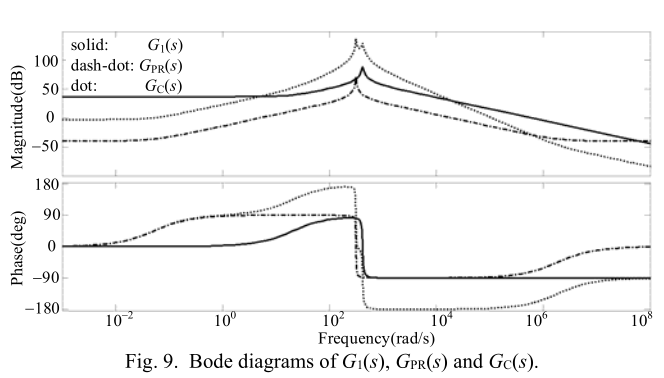#### B. 电容电流反馈

$G _ { 2 } ( s ) = \frac { i _ { o } ( s ) } { v _ { r } ( s ) } = \frac { K _ { pwm } ( s R _ { s } C _ { s } + 1 ) } { s ^ { 2 } R _ { s } L _ { o } ( C _ { o } + C _ { s } ) + s ( L _ { o } + K _ { pwm } H _ { i } R _ { s } C _ { o } ) + R _ { s } }\tag{13}$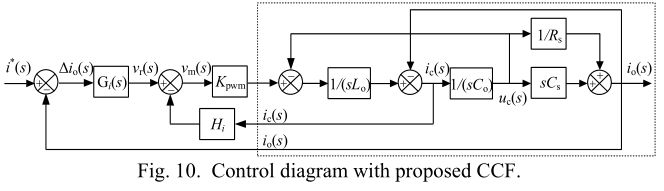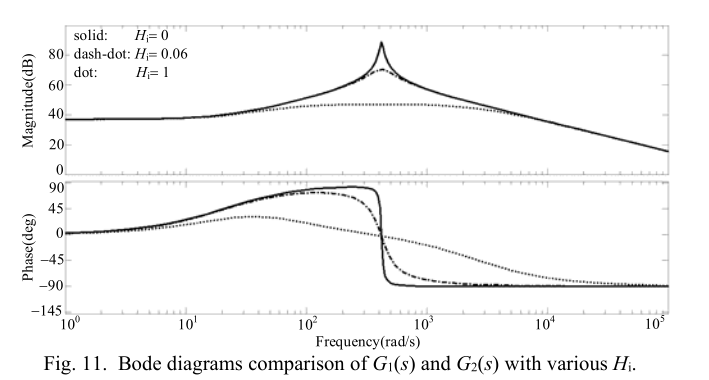#### C. 电流调节器

$G _ { PR } ( s ) = k _ { p _ { - } PR } + \frac { 2 k _ { r } \omega _ { i } S } { s ^ { 2 } + 2 \omega _ { i } S + \omega _ { 0 } ^ { 2 } }\tag{14}$

$G _ { PI } ( s ) = k _ { p _ { - } PI } + \frac { k _ { i } } { s }\tag{15}$

$G _ { i } ( s ) = G _ { PR } ( s ) G _ { PI } ( s )\tag{16}$ $G _ { t } ( s ) = G _ { PR } ( s ) G _ { PI } ( s ) G _ { 2 } ( s )\tag{17}$

### 4. 控制参数设计

#### A. PWM 约束

$H _ { i } \leq \frac { 4 f _ { sw } L _ { o } } { K _ { pwm } }\tag{18}$

#### B. PI控制器

$\frac { k _ { i } } { k _ { p _{ —PI} } } \ll \omega _ { c }\tag{19}$

$k _ { i } \ll \omega _ { c }\tag{20}$

#### C. 交叉频率$\omega_c$

$| G _ { i } ( j \omega _ { c } ) | = | G _ { PR } ( j \omega _ { c } ) G _ { PI } ( j \omega _ { c } ) | = k _ { p _ { - } PR }\tag{21}$

$| G _ { t } ( j \omega _ { c } ) | = 1\tag{22}$

$| \frac { k _ { p , PR } K _ { pwm } ( 1 + j \omega _ { c } R _ { s } C _ { s } ) } { R _ { s } [ 1 - \omega _ { c } ^ { 2 } L _ { 0 } ( C _ { o } + C _ { s } ) ] + j \omega _ { c } ( L _ { o } + K _ { pwm } H _ { i } R _ { s } C _ { o } ) } | = 1\tag{23}$

$| \frac { k _ { p _ { DR } } ( 1 - j d \frac { \omega _ { 0 } } { \omega _ { c } } ) } { \frac { d \omega _ { 0 } L _ { o } } { K _ { pwm } } + H _ { i } \frac { C _ { o } } { C _ { s } } - j \frac { 1 } { K _ { pwm } } ( \frac { 1 } { \omega _ { c } C _ { s } } - \omega _ { c } L _ { o } ) } | = 1\tag{24}$

$k _ { p _ { - } PR } ^ { 2 } - H _ { i } ^ { 2 } ( \frac { C _ { o } } { C _ { s } } ) ^ { 2 } = ( \frac { \omega _ { c } L _ { o } } { K _ { pwm } } ) ^ { 2 }\tag{25}$

$k _ { p _ { - } PR } = \frac { \omega _ { c } L _ { o } } { K _ { pwm } }\tag{26}$

$\frac { \omega _ { c } L _ { o } } { K _ { pwm } } \frac { K _ { pwm } } { R _ { s } } = \frac { \omega _ { c } L _ { o } } { R _ { s } }\tag{27}$

#### D. 稳态误差和稳定裕度约束

$E _ { i } = | \frac { i ^ { * } ( j \omega _ { 0 } ) - i _ { o } ( j \omega _ { 0 } ) } { i ^ { * } ( j \omega _ { 0 } ) } | \tag{28}$

$E _ { i } = | 1 - \frac { G _ { t } ( j \omega _ { 0 } ) } { 1 + G _ { t } ( j \omega _ { 0 } ) } | = | \frac { 1 } { 1 + G _ { t } ( j \omega _ { 0 } ) } | \tag{29}$

$\frac { 1 } { E _ { i } } = | 1 + \frac { ( k _ { p _ { - } PR } + k _ { r } ) K _ { pwm } } { d \omega _ { 0 } L _ { o } + K _ { pwm } H _ { i } C _ { o } / C _ { s } - j ( 1 / \omega _ { 0 } C _ { s } - \omega _ { 0 } L _ { o } ) } | \tag{30}$

$\frac { k _ { p _ { LR } } + k _ { r } } { H _ { i } } \approx \frac { C _ { o } } { C _ { s } E _ { i } } \tag{31}$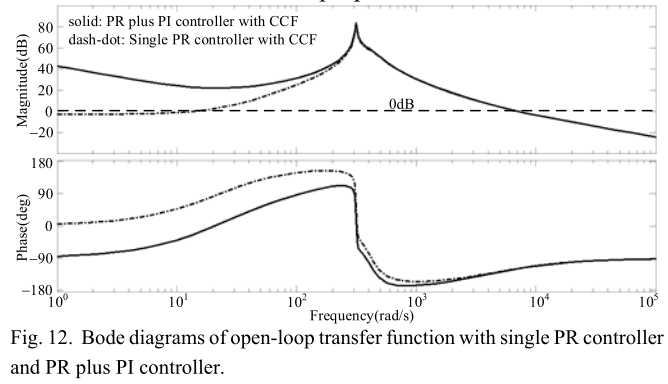$G _ { PR } ( j \omega _ { c } ) = k _ { p _ { - } PR } + 2 k _ { r } \omega _ { i } / s \tag{32}$

$P M = 180 ^ { \circ } + \angle G _ { PR } ( j \omega _ { c } ) + \angle G _ { 2 } ( j \omega _ { c } ) \tag{33}$

$\frac { k _ { r } } { k _ { p _ { p _ { PR } } } } \geq \frac { \omega _ { c } ( \omega _ { c } L _ { o } C _ { s } + K _ { pwm } C _ { o } H _ { i } \operatorname { tan } P M ) } { 2 \omega _ { i } ( \omega _ { c } L _ { o } C _ { s } \operatorname { tan } P M - K _ { pwm } C _ { o } H _ { i } ) } \tag{34}$

### 5. 参数设计及实验结果

#### A. 参数设计

PI和复合控制器的比较Bode图也显示在图13中，两者都没有CCF。 显然，前者具有更大的分频频率和相位裕度，比拟议的具有更快的响应和更好的稳定性。 但是，它不能有效地抑制输出电流的谐波。 没有CCF的复合控制器遭受负载谐振的the180°相移，因此由于负增益裕量而不稳定。建议的接地系统的额定电压和额定电流也需要讨论。 从系统拓扑结构来看，额定电压与（1）和（2）中所示的中性点到地电压有关。 接地系统的补偿电流由分布参数的不对称性确定，如（4）所示。 从这些表达式可以看出，最坏的情况发生在两相开路条件下。 在这种情况下，中性点电压上升到线对中性点电压，并且补偿后的电流基准达到系统充电电流的1/3。

#### B. 实验结果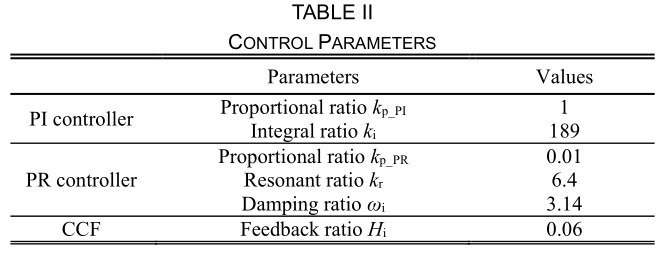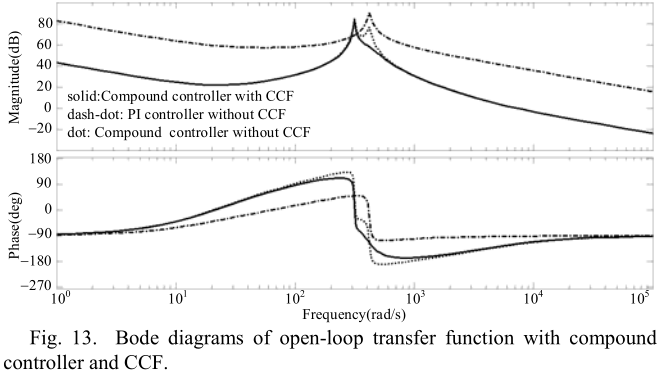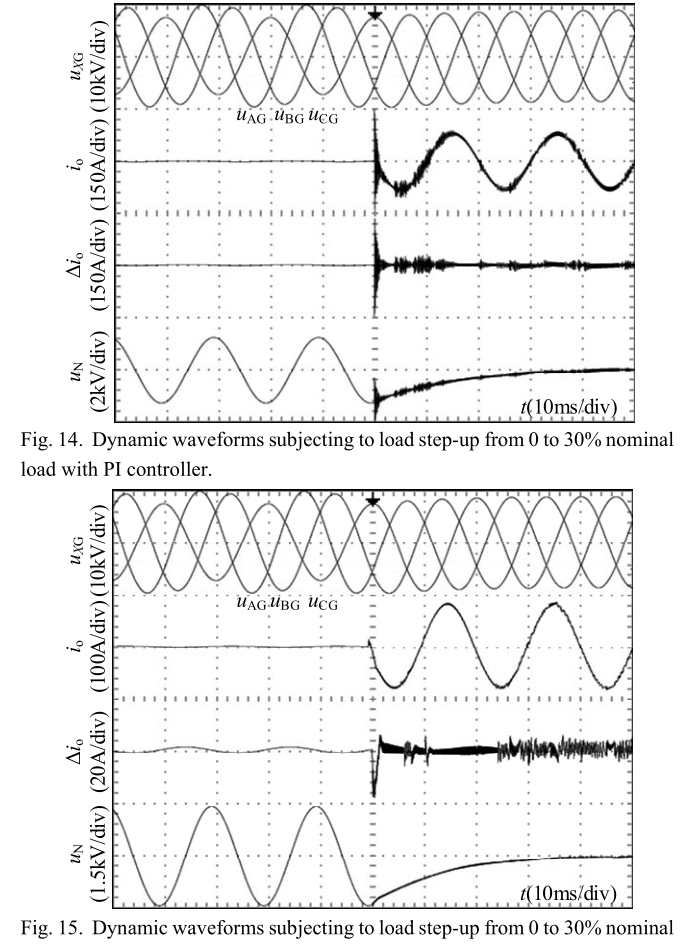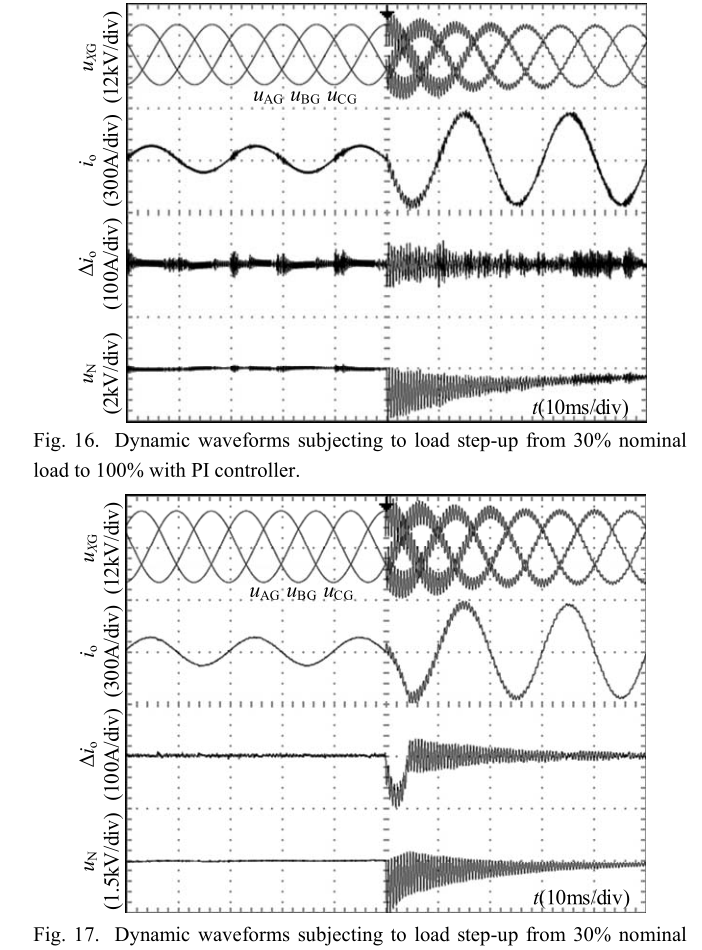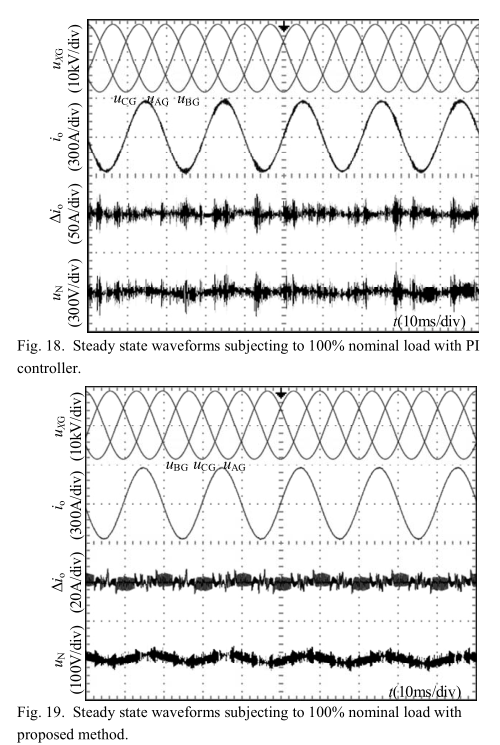### 6. 结论

#### 附录

$G _ { 2 } ( j \omega _ { c } ) = \frac { K _ { pwm } ( 1 - j d \frac { \omega _ { 0 } } { \omega _ { c } } ) } { \frac { L _ { o } + K _ { pwm } H _ { i } R _ { s } C _ { o } } { R _ { s } C _ { s } } + j ( \omega _ { c } L _ { o } - \frac { 1 } { \omega _ { c } C _ { s } } ) } \tag{A.1}$

$\angle G _ { 2 } ( j \omega _ { c } ) = - \operatorname { arctan } \frac { \omega _ { c } L _ { o } R _ { s } C _ { s } } { L _ { o } + K _ { pwm } H _ { i } R _ { s } C _ { o } } \tag{A.2}$

$P M = 180 ^ { \circ } - \operatorname { arctan } \frac { 2 k _ { r } \omega _ { i } } { k _ { p _ { PRR } } \omega _ { c } } - \operatorname { arctan } \frac { \omega _ { c } L _ { o } R _ { s } C _ { s } } { L _ { o } + K _ { pwm } H _ { i } R _ { s } C _ { o } } \tag{A.3}$

$\operatorname { rctan } \frac { 2 k _ { r } \omega _ { i } } { k _ { p , PR } \omega _ { c } } + \operatorname { arctan } \frac { \omega _ { c } L _ { o } R _ { s } C _ { s } } { K _ { pwm } H _ { i } R _ { s } C _ { o } } > 180 ^ { \circ } - PM \tag{A.4}$

$\frac { k _ { r } } { k _ { p _ { pR } } } \geq \frac { \omega _ { c } ( \omega _ { c } L _ { o } C _ { s } + K _ { pwm } C _ { o } H _ { i } \operatorname { tan } P M ) } { 2 \omega _ { i } ( \omega _ { c } L _ { o } C _ { s } \operatorname { tan } P M - K _ { pwm } C _ { o } H _ { i } ) } \tag{A.5}$

### 参考文献

 IEEE Recommended Practice for Grounding of Industrial and Commercial Power Systems, IEEE Std. 142-2007, 2007.

 L. J. Kingrey, R. D. Painter, and A. S. Locker, “Applying High-Resistance Neutral Grounding in Medium-Voltage Systems,” IEEE Trans. Ind. App., vol. 47, no. 3, pp. 1220– 1231, May 2011.

 X. Zeng, Y. Xu, and Y. Wang, “Some novel techniques for insulation parameters measurement and Petersen-coil control in distribution networks,” IEEE Trans. Ind. Electron., vol. 57, no. 4, pp. 1445–1451, Apr.2010.

 A. Kalyuzhny, “Analysis of Temporary Overvoltages During Open-Phase Faults in Distribution Networks With Resonant Grounding,” IEEE Trans.Power Del., vol.30, no.1, pp.420-427, Feb. 2015.

 X. Lin, J. Huang, and S. Ke, “Faulty Feeder Detection and Fault SelfExtinguishing by Adaptive Petersen Coil Control,” IEEE Trans. Power Del., vol.26, no.2, pp.1290-1291, Apr. 2011.

 M. Brenna, E. D. Berardinis, L. D. Carpini, P. Paulon, P. Petroni, G. Sapienza, G. Scrosati, and D. Zaninelli, “Petersen Coil Regulators Analysis Using a Real-Time Digital Simulator,” IEEE Trans. Power Del., vol.26, no.3, pp.1479-1488, July 2011.

 X. Zeng, K. K. Li, W. L. Chan, S. Su, and Y. Wang, “Ground-Fault Feeder Detection With Fault-Current and Fault-Resistance Measurement in Mine Power Systems,” IEEE Trans. Ind. App., vol. 44, no. 2, pp. 424– 429, Mar.2008.

 J. Tian, Q. Chen, L. Cheng, and Y. Zhang, “Arc-suppression coil based on transformer with controlled load,” IET Elect. Power App., vol.5, no.8, pp.644-653, September 2011.

 X. Chen, B. Chen, C. Tian, J. Yuan, and Y. Liu, “Modeling and Harmonic Optimization of a Two-Stage Saturable Magnetically Controlled Reactor for an Arc Suppression Coil,” IEEE Trans. Ind. Electron., vol.59, no.7, pp.2824-2831, July 2012

 D. Paul, P. E. Sutherland, and S. A. R. Panetta, “A Novel Method of Measuring Inherent Power System Charging Current,” IEEE Trans. Ind.App., vol. 47, no. 6, pp. 3230– 3240, Nov. 2011.

 D. Dong, T. Thacker, R. Burgos, F. Wang, and D. Boroyevich, “On zero steady-state error voltage control of single-phase PWM inverters with different load types,” IEEE Trans. Power Electron., vol. 26, no. 11, pp.3285– 3297, Nov. 2011.

 Y. Tang, P. C. Loh, P. Wang, F. H. Choo, F. Gao, and F. Blaabjerg, “Generalized design of high performance shunt active power filter with output LCL filter,” IEEE Trans. Ind. Electron., vol. 59, no. 3, pp. 1443– 1452, Mar. 2012.

 F. Liu, Y. Zhou, S. Duan, J. Yin, B. Liu, and F. Liu, “Parameter Design of a Two-Current-Loop Controller Used in a Grid-Connected Inverter System With LCL Filter,” IEEE Trans. Ind. Electron., vol. 56, no. 11, pp.4483–4491, Nov. 2009.

 G. Shen, X. Zhu, J. Zhang, and D. Xu, “A New Feedback Method for PR Current Control of LCL-Filter-Based Grid-Connected Inverter,” IEEE Trans. Ind. Electron., vol. 57, no. 6, pp. 2033–2041, Jun. 2010.

 X. Dong and S. Shi, “Identifying Single-Phase-to-Ground Fault Feeder in Neutral Noneffectively Grounded Distribution System Using Wavelet Transform,” IEEE Trans. Power Del., vol. 23, no. 4, pp. 1829-1837, Oct.2008.

 A. Kuperman, “Proportional-Resonant Current Controllers Design Based on Desired Transient Performance,” IEEE Trans. Power Electron., vol.30, no. 10, pp. 5341–5345, Oct. 2015.

 R. Peña-Alzola, M. Liserre, F. Blaabjerg, R. Sebastián, J. Dannehl, and F. W. Fuchs, “Analysis of the Passive Damping Losses in LCL-Filter-Based Grid Converters,” IEEE Trans. Power Electron., vol. 28, no. 6, pp. 2642– 2646, Jun. 2013.

 W. Wu, Y. He, T. Tang, and F. Blaabjerg, “A New Design Method for the Passive Damped LCL and LLCL Filter-Based Single-Phase Grid-Tied Inverter,” IEEE Trans. Ind. Electron., vol. 60, no. 10, pp. 4339–4350, Oct.2013.

 M. Liserre, R. Teodorescu, and F. Blaabjerg, “Stability of photovoltaic and wind turbine grid-connected inverters for a large set of grid impedance values,” IEEE Trans. Power Electron., vol. 21, no. 1, pp. 263– 272, Jan. 2006.

 Erickson and D. Maksimovic, Fundamentals of Power Electronics, 2nd ed. Norwell, MA, USA: Kluwer, 2001, pp. 331–408.

 C. Bao, X. Ruan, X. Wang, W. Li, D. Pan, and K. Weng, “Step-by-Step Controller Design for LCL-Type Grid-Connected Inverter with Capacitor–Current-Feedback Active-Damping,” IEEE Trans. Power Electron., vol. 29, no. 3, pp. 1239 - 1253, Mar. 2014.